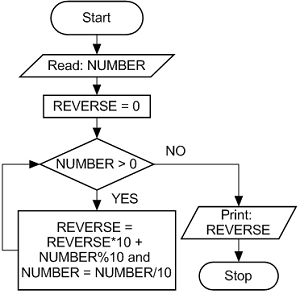# Reverse a number in c++ || C++ Program to Reverse a Number->

Write a program to reverse the digits of an integer.
```Input : num = 12345
Output: 54321

Input : num = 876
Output: 678```
``
```Algorithm:Input:  num
(1) Initialize rev_num = 0
(2) Loop while num > 0
(a) Multiply rev_num by 10 and add remainder of num
divide by 10 to rev_num
rev_num = rev_num*10 + num%10;
(b) Divide num by 10
(3) Return rev_num```